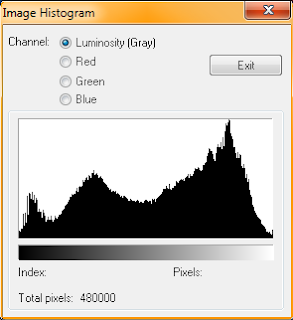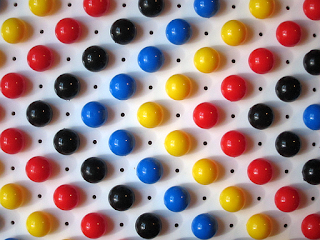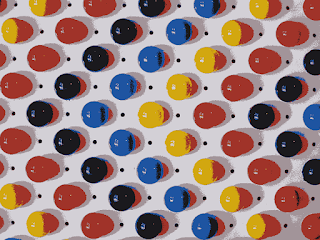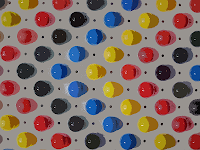## Sunday, June 16, 2013

### Image segmentation with K-means algorithm - Java implementation

K-means algorithm is a well known clustering algorithm and it is described in many papers and online texts. But even after reading many of these said documents, I was confused and still had many questions in my mind.

I was wondering questions like:
• What are the K cluster centers in digital image case?
• What is the minimal distance function when comparing clusters?
I left these questions in my mind for few days, and finally on one morning I realized what the K-means algorithm means in a context of digital image segmentation. Segmentation in this case means "finding the continuous areas where the color and intensity is similar to adjacent pixels".

I will try to explain the questions above in this post.

K-means algorithm for digital images in a nut shell
1. create k clusters, k being the cluster count and the "center" being a color value
2. for each pixel, find the cluster which has minimal distance to the pixel
3. if the pixel was already in other cluster, remove the pixel from other cluster and add it to a new cluster
4. when pixel is added to a cluster, adjust the cluster center color value by adding the pixel colors to it, remove the pixel color values from the old cluster
5. loop back to 2. until there are no pixels that are changing clusters
The algorithm works like magic! By adding and removing the pixels from clusters, the clusters will transform automatically close to the optimal solution (but the result may not be the optional solution).

K-means cluster centers in digital images

In K-means algorithm you have to decide how many clusters you will have in your image. The simplest case is that the cluster count means the color count and the cluster centers are the color values. In other words, if you have an RGB image with millions of colors, after K-means clustering with value 20, you will have the image converted to a version which only has 20 colors.

Take a look at the histograms below. Now, choosing the value 20 and running the K-means algorithm on the image, the colors are reduced to 20 colors. The histogram after running the algorithm is on below.Histogram from original imageHistogram after K-means algorithm

In my algorithm implementation, I am choosing the cluster centers always in the same way, so the result for the same original image is always the same. But if you choose the cluster centers randomly, you will end up having slightly different results.

Distance function when comparing clusters

If you chose to continue with simplest case where cluster centers are simply color values, the compare function is also simple. Just calculate how far, or what is the distance, between the pixel you are working with and the cluster center color is.

When working with gray level images the distance calculation is easy, but for color images you need to split the RGB values to individual red, green and blue values. Then calculate the difference between each pixel color and cluster color and finally average the result.

My implementation

I have implemented two different approaches for adding pixels to the clusters. I have named them "continuous" and "iterative" clustering.

In "continuous clustering" I am adding and removing pixels to and from clusters for each pixel if necessary, and I am also counting the cluster center again after each added or removed pixel. The algorithm finds solution faster than the iterative clustering.

In "iterative clustering" I am adding all the pixels to clusters first, and after that I am calculating the new cluster centers.

There are small differences between the results of these two methods. I _guess_ the differences are coming from integer rounding.

Sample imagesThe original test imageResult of continuous clustering (took 6 loops, 816 milliseconds)Result of iterative clustering (took 62 loops, 9158 milliseconds)

Java implementation

 package popscan; import java.awt.image.BufferedImage; import java.io.File; import java.util.Arrays; import javax.imageio.ImageIO; public class KMeans { BufferedImage original; BufferedImage result; Cluster[] clusters; public static final int MODE_CONTINUOUS = 1; public static final int MODE_ITERATIVE = 2; public static void main(String[] args) { if (args.length!=4) { System.out.println("Usage: java popscan.KMeans" + " [source image filename]" + " [destination image filename]" + " [clustercount 0-255]" + " [mode -i (ITERATIVE)|-c (CONTINUOS)]"); return; } // parse arguments String src = args; String dst = args; int k = Integer.parseInt(args); String m = args; int mode = 1; if (m.equals("-i")) { mode = MODE_ITERATIVE; } else if (m.equals("-c")) { mode = MODE_CONTINUOUS; } // create new KMeans object KMeans kmeans = new KMeans(); // call the function to actually start the clustering BufferedImage dstImage = kmeans.calculate(loadImage(src), k,mode); // save the resulting image saveImage(dst, dstImage); } public KMeans() {    } public BufferedImage calculate(BufferedImage image, int k, int mode) { long start = System.currentTimeMillis(); int w = image.getWidth(); int h = image.getHeight(); // create clusters clusters = createClusters(image,k); // create cluster lookup table int[] lut = new int[w*h]; Arrays.fill(lut, -1); // at first loop all pixels will move their clusters boolean pixelChangedCluster = true; // loop until all clusters are stable! int loops = 0; while (pixelChangedCluster) { pixelChangedCluster = false; loops++; for (int y=0;y>16&0x000000FF; int g = rgb>> 8&0x000000FF; int b = rgb>> 0&0x000000FF; red = r; green = g; blue = b; this.id = id; addPixel(rgb); } public void clear() { red = 0; green = 0; blue = 0; reds = 0; greens = 0; blues = 0; pixelCount = 0; } int getId() { return id; } int getRGB() { int r = reds / pixelCount; int g = greens / pixelCount; int b = blues / pixelCount; return 0xff000000|r<<16|g<<8|b; } void addPixel(int color) { int r = color>>16&0x000000FF; int g = color>> 8&0x000000FF; int b = color>> 0&0x000000FF; reds+=r; greens+=g; blues+=b; pixelCount++; red   = reds/pixelCount; green = greens/pixelCount; blue  = blues/pixelCount; } void removePixel(int color) { int r = color>>16&0x000000FF; int g = color>> 8&0x000000FF; int b = color>> 0&0x000000FF; reds-=r; greens-=g; blues-=b; pixelCount--; red   = reds/pixelCount; green = greens/pixelCount; blue  = blues/pixelCount; } int distance(int color) { int r = color>>16&0x000000FF; int g = color>> 8&0x000000FF; int b = color>> 0&0x000000FF; int rx = Math.abs(red-r); int gx = Math.abs(green-g); int bx = Math.abs(blue-b); int d = (rx+gx+bx) / 3; return d; } } }

 Check out my blogpost about watershed segmentation: http://popscan.blogspot.fi/2014/04/watershed-image-segmentation-algorithm.html

## Saturday, June 8, 2013

### Color image segmentation by thresholding with Java

You may need the image segmentation tool for various image analysis tasks. Different tasks may also need specialized segmentation algorithm, for example the segmentation process may discard all green pixels and keep yellow and red, or if you know the object you are trying to detect contains only blue.

It may be difficult to adjust one general segmentation algorithm for special cases, so you may need to tweak the segmentation code itself, not just the parameters.

I will present here one variation from the simplest segmentation algorithm, segmentation by thresholding, implemented in pure Java.

More sophisticated methods exist, and you should use them if your problem requires so.

In my opinion, this thresholding function gives you a nice starting point when starting to make experiments with segmentation. From the command line, you can only adjust the threshold level, int value 0 - 255, but from the source you can change the connectivity testing and balance the color thresholding if needed.

Example images:The original photoSegmented with threshold value 20Segmented with threshold value 40Segmented with threshold value 60Segmented with threshold value 80Segmented with threshold value 100Segmented with threshold value 120

Feel free to copy the code and adapt it to your own source and frameworks.

Improvements:
I will make an version from the algorithm where the segment color is averaged from the pixels in the segment. In this version, the color is the color which is the "seed" pixel color.

The algorithm in pseudo code:

for each pixel in image
if pixel is not in segment
create new segment
add the pixel as new "seed" point to list of candidates
while segment has candidate points
remove first point from the list of candidates
if the first candidate point is within threshold limit

add the first candidate point to the segment
add neighbor pixel above to the candidate list
add neighbor pixel below to the candidate list
add neighbor pixel right to the candidate list
add neighbor pixel left  to the candidate list

This kind of algorithm must use the candidate list and while loop instead of recursion, because in the worst case, the whole image (its pixels) belong to one segment. In that case, when using recursion, you will most likely see "StackOverflowError" with big images.

The Java implementation: